• 刚开始接触循环群不容易理解，不妨以模6加法群&lt;Z6，+&gt;入手，来认识循环群的特点。...取其中的元素1，不停地对自身进行模6加法，即对本身进行幂运算。 可得： 1^1=1 1^2=1+1=2 1^3=1+1+1=3 1^4=1+...
 刚开始接触循环群不容易理解，不妨以模6加法群<Z6，+>入手，来认识循环群的特点。

首先，循环群，顾名思义，cycle group即带有循环的意思。怎么个循环法呢？

我们看<Z6，+>中的元素{0,1,2,3,4,5}。取其中的元素1，不停地对自身进行模6加法，即对本身进行幂运算。

可得：

1^1=1

1^2=1+1=2

1^3=1+1+1=3

1^4=1+1+1+1=4

1^5=1+1+1+1+1=5

1^6=1+1+1+1+1+1=0（模6加法意义下）

1^7=1+1+1+1+1+1+1=1（模6加法意义下）

……

如上对1不断幂运算，可见两个现象：

1、可以遍历所有的元素，也可以说，我们仅用元素1就能生成所有的元素，这就是循环群里的生成元的概念。

2、幂运算的结果就是123450123450123450这样不断的循环，这就是循环群名字由来。

现在有了感性认识，可以对循环群用准确的数学语言定义，就是：

若存在a∈G使得G=<a>,则称G是循环群，称a为G的生成元。

现在，我们继续思考，如果对其它元素进行不断的幂运算呢，会出现什么结果？

经过不断的幂运算，我们发现

元素0形成的结果只有0，可写成，结果集合为{0}，

元素2、4形成的结果是一样的，结果集合为{0,2,4}，

元素3形成的结果集合为{0,3}，

元素1、5形成的结果为{0,1,2,3,4,5}，

可见，不同的元素，有的形成的结果不同，有的却相同。我们可以按照他们生成的结果来将他们划分为不同的群体。

对于元素1、5，他们都能生成所有元素，所以他们两个元素不仅证明了这个群是循环群，还说明他们都是循环群的生成元。

他们生成了{0,1,2,3,4,5}这个子群（或者说群本身，也叫平凡子群）并且他们都是6阶元素，所谓6阶，就是a^6=e=0(幺元，或称单位元，这个群的单位元是0)。6阶也是这个群的阶数。

对于元素2、4，他们生成了子群{0,2,4}，他们都是3阶元素。

对于元素3，生成了子群{0,3}，他是2阶元素。

对于元素0，生成了子群{0}，他是1阶元素。

通过对上面的观察，我们又看出一些规律，就是：

1、n阶元素生成的子群中具有n个元素

2、一个n阶群，他具有p个不同类型的生成子群，p是n的正因子个数，比如本例中6的正因子有1,2,3,6共四个。

3、一个n阶群，他的生成元个数是与小于n且与n互为素数的个数。本例中，小于6且与6互素的数是1、5，共两个，所以这个群的生成元就正好2个。

以上规律均可证明，有兴趣可以自己进行证明，深入学习。
展开全文• Z6={0,1,2,3,4,5}，模 6 加法的构成的群中，群的阶=？...取其中的元素1，不停地对自身进行模6加法，即对本身进行幂运算。 可得： 5=5 5+5=10 5+5+5=15 5+5+5+5=20 5+5+5+5+5=25 5+5+5+5+5+5=30 故...
Z6={0,1,2,3,4,5}，模 6 加法的构成的群中，群的阶=？， 元素 5 的阶 |5 |＝？ 。
我们知道，群的阶等于元素个数6，
我们看<Z6，+>中的元素{0,1,2,3,4,5}。取其中的元素1，不停地对自身进行模6加法，即对本身进行幂运算。
可得：
5=5
5+5=10
5+5+5=15
5+5+5+5=20
5+5+5+5+5=25
5+5+5+5+5+5=30
故|5|=6
考虑大数字不好计算，让我们通过编程找规律。
/*
模n加法，群的阶=n，元素的阶=最少几次方（几次相加）=本身。
下面来求元素的阶
*/

#include<iostream>
#include<iomanip>
using namespace std;
int as(int a)
{
int i, j = 0;
for (i = 2; i < a; i++)
if (a % i == 0)
{
j = 1; break;
}
return j;
}
int main()
{
int x, y, i;
int a = 15, b = 4;//a为元素个数和模a加法中的a.b为预留空间
for (i = 0; i < a; i++)
cout << setw(b) << i;
for (y = 2; y <= a; y++)
//	if(as(y))
{
cout << endl << setw(b) << y;
for (x = 1; x < y; x++)
for (i = 2; i < y + 2; i++)
if ((i * x) % y == x)
{
cout << setw(b) << i-1; break;
}
}
return 0;
}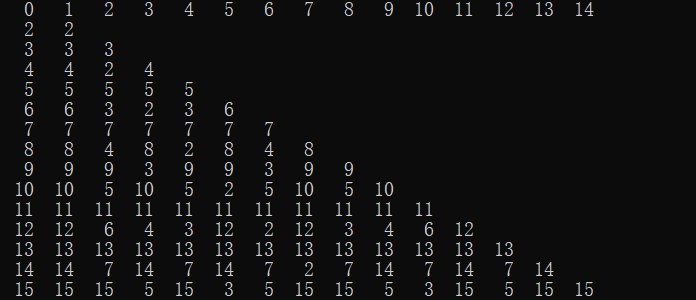运行结果最上方一行为元素，最左方一列为元素个数，其它均为元素的阶。
不难看出：元素的阶=n/（n与元素的最大公约数）
|5|=6/（6与5最大公约数=1）=6


展开全文c++
• 就是能表示的最大数字。 1）将 1 1-1-1 转换为补码，1 01011， 1 01011 + 0 11011 = 0 00110 化为十进制：6 2）将1 10110 转换为补码，1 01010，将1 00001 转换为补码，1 11111 1 01010 + 1 11111 = 1 01001...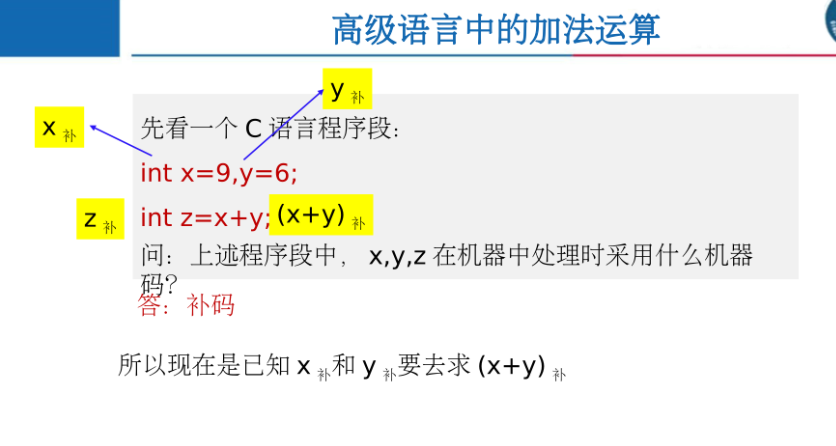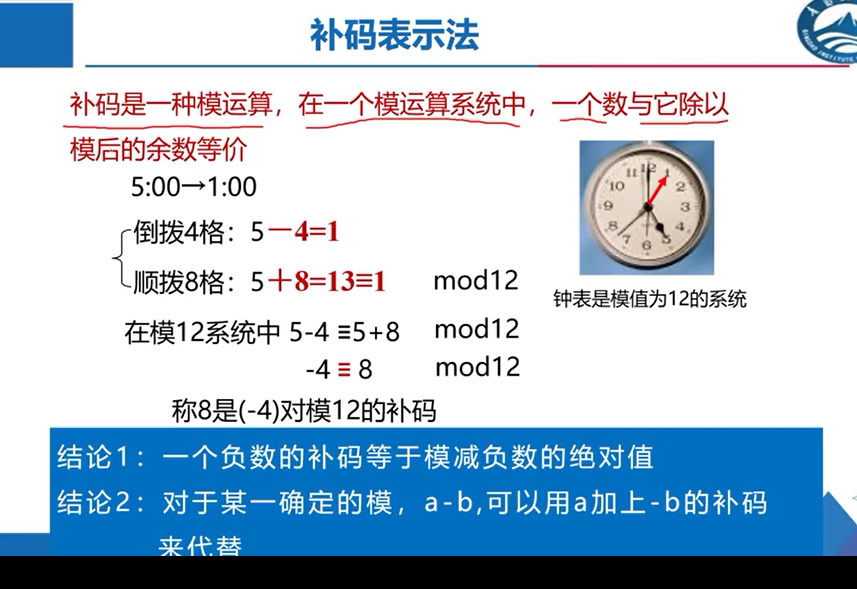模就是能表示的最大数字。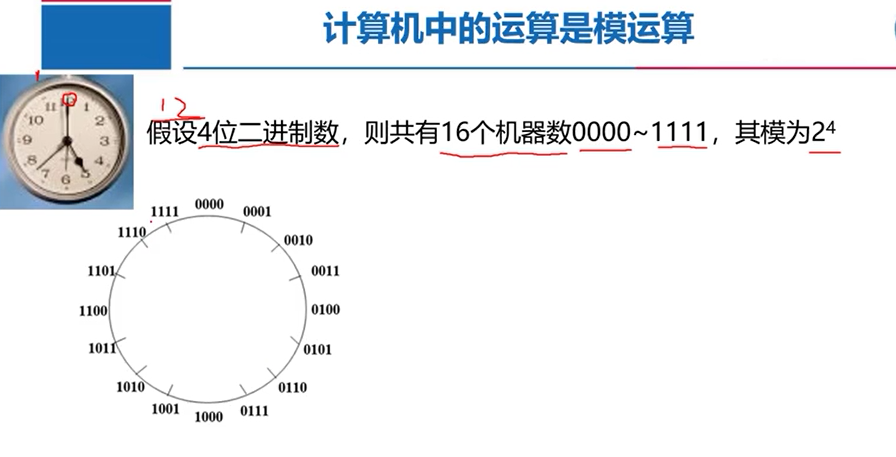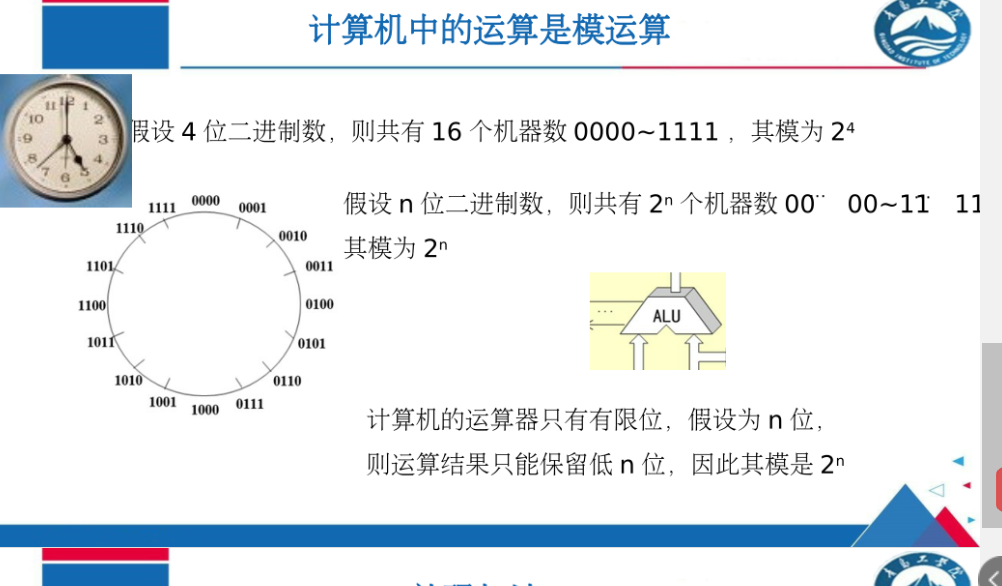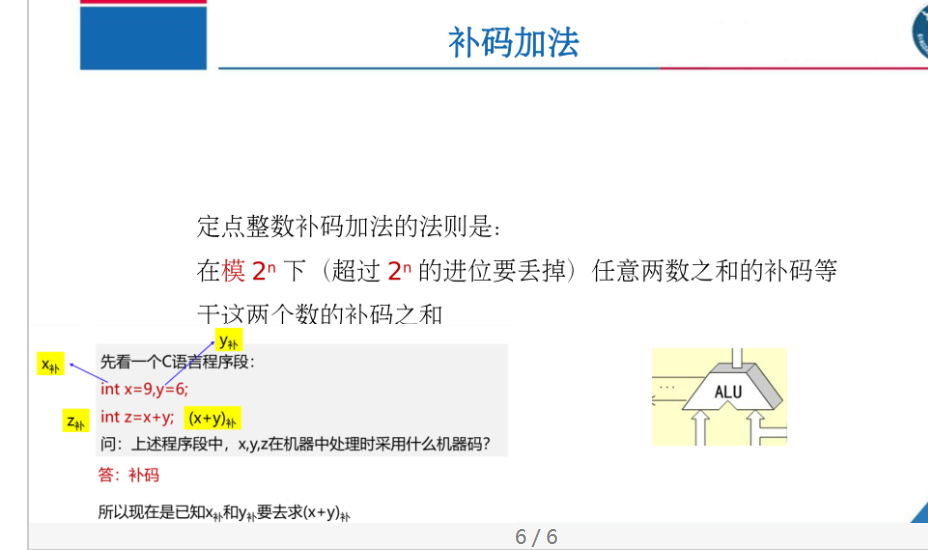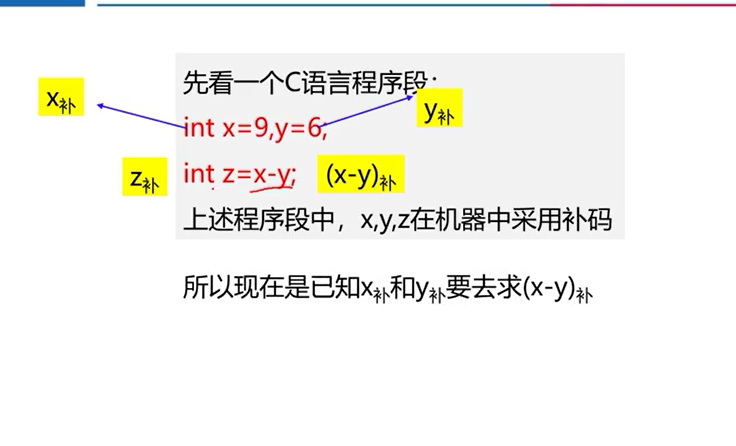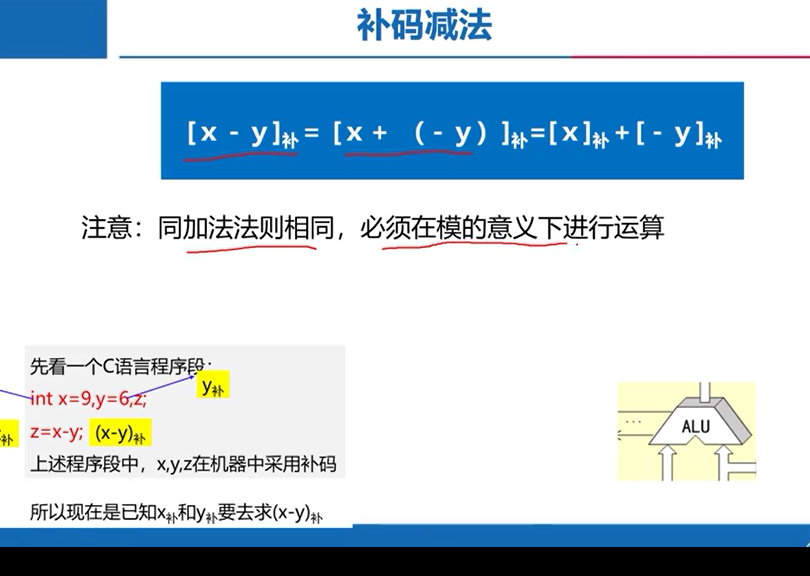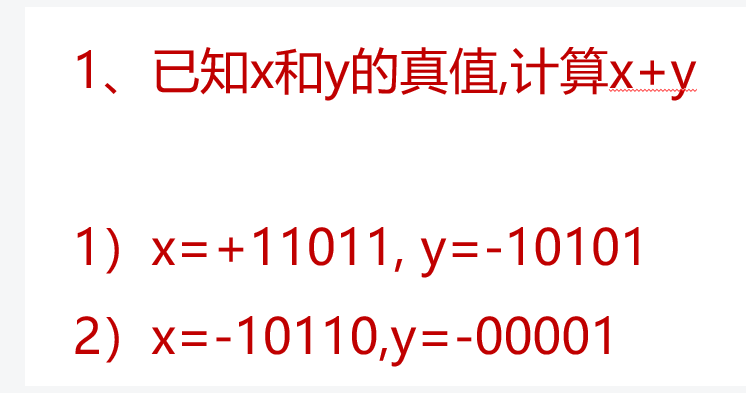1）将 1 10101 转换为补码，1 01011，
1 01011 + 0 11011 = 0 00110 化为十进制：6
2）将1 10110 转换为补码，1 01010，将1 00001 转换为补码，1 11111
1 01010 + 1 11111 = 1 01001 转换为源码1 10111化为十进制：-23
减法运算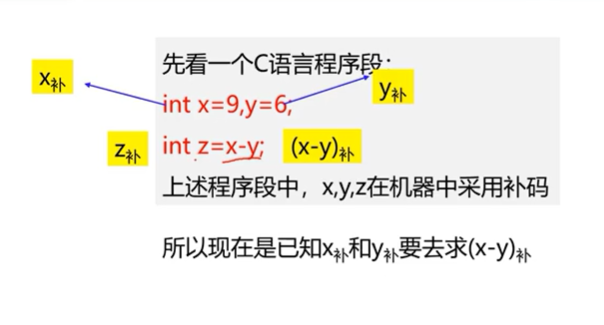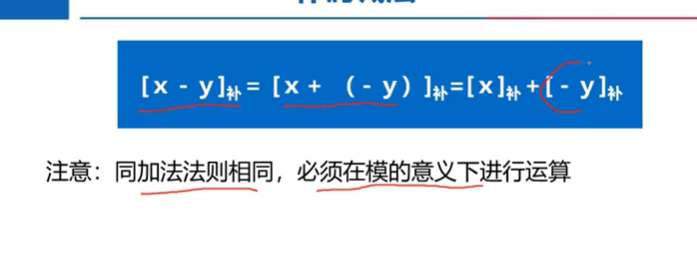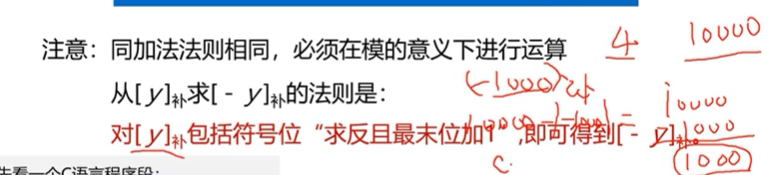5.2.1溢出判断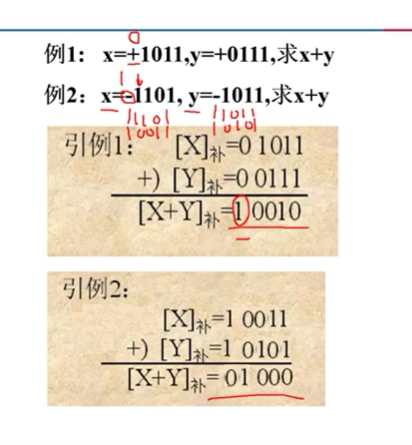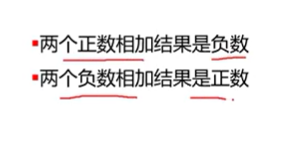这种情况叫做溢出。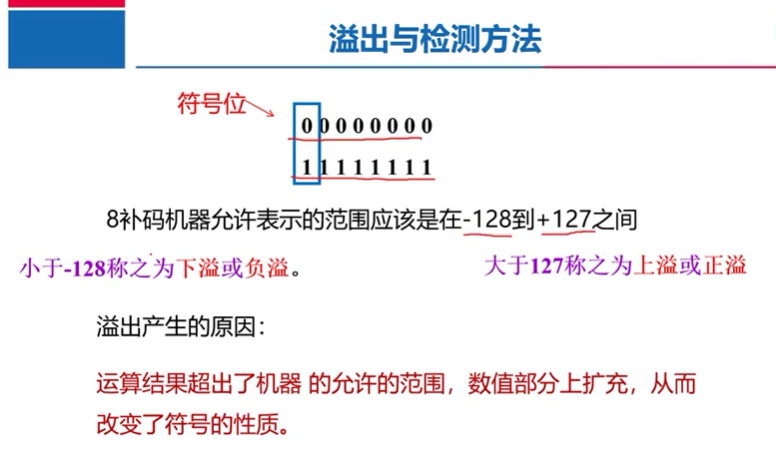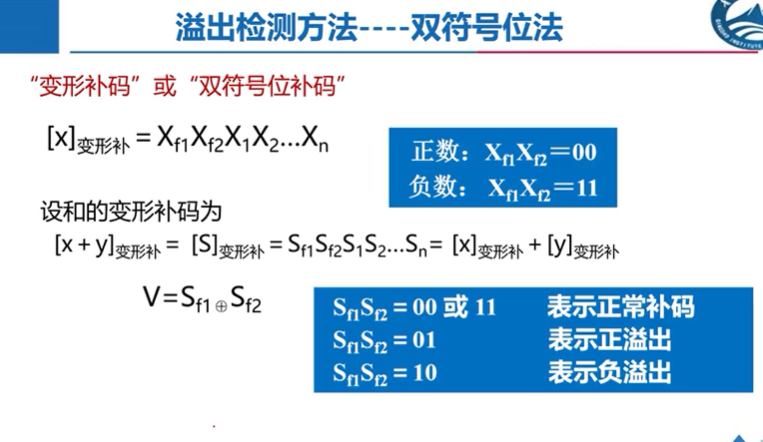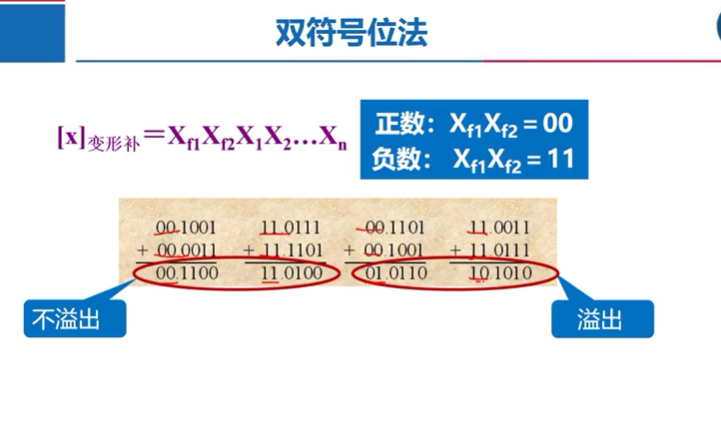优点是直观简单，缺点是符号位需要多占一位。数据表示的范围就会变小。
单符号位法可以有效解决这个问题。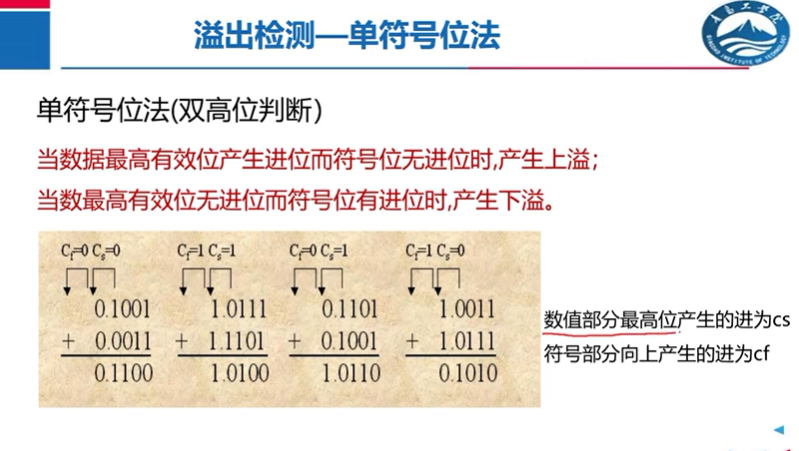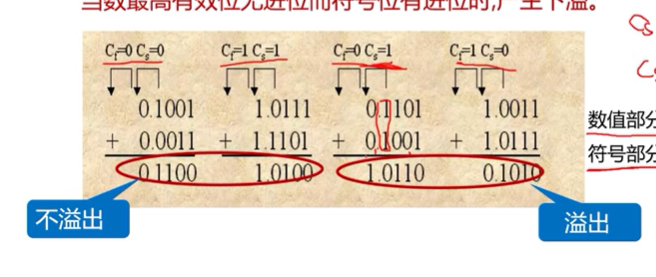cs和cf的值相等就是正常，不相等就是溢出。
5.2.2行波进位加减器
行波进位加减器的组成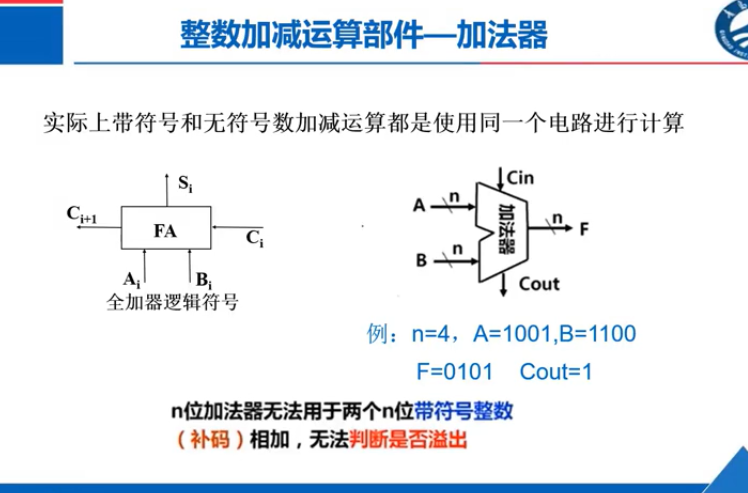左边的是一位全加器，右边的是n位加法器，n位数与n位数相加。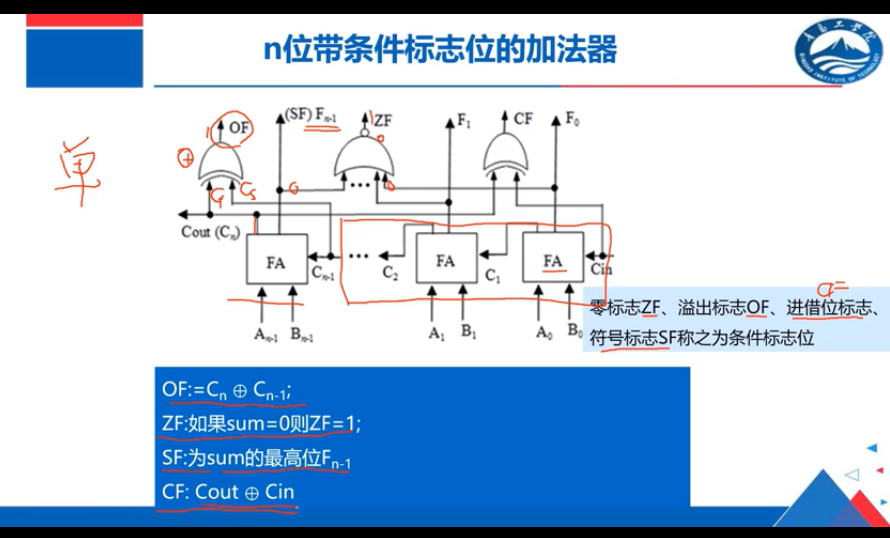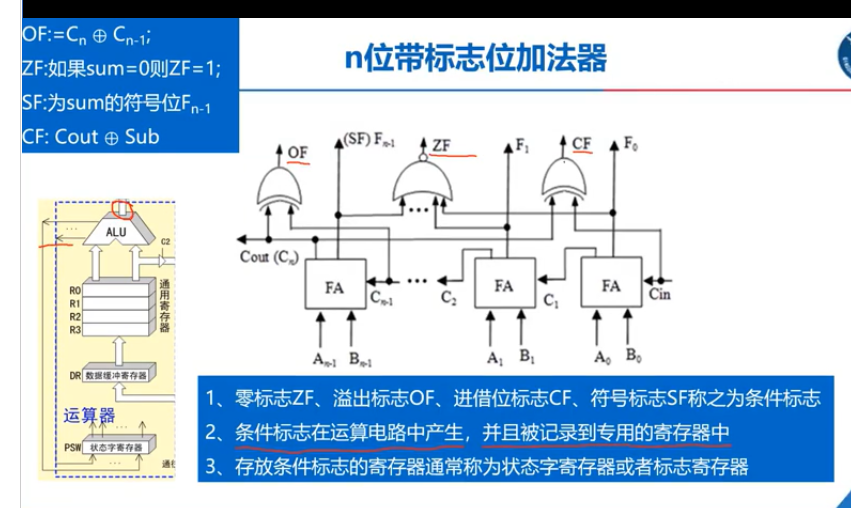OF溢出标志位是Cn-1与Cout做异或，也就是数值的进位和符号位做异或。
ZF零标志位就是如果每一位的输出全是0，那么最后ZF就是1。
SF符号标志位就是符号运算的结果。
CF进借位标志位
将加法器改进一下就可以进行减法运算。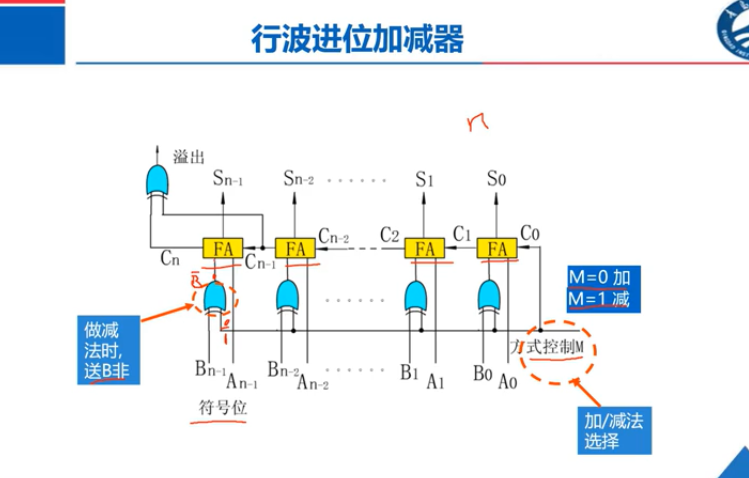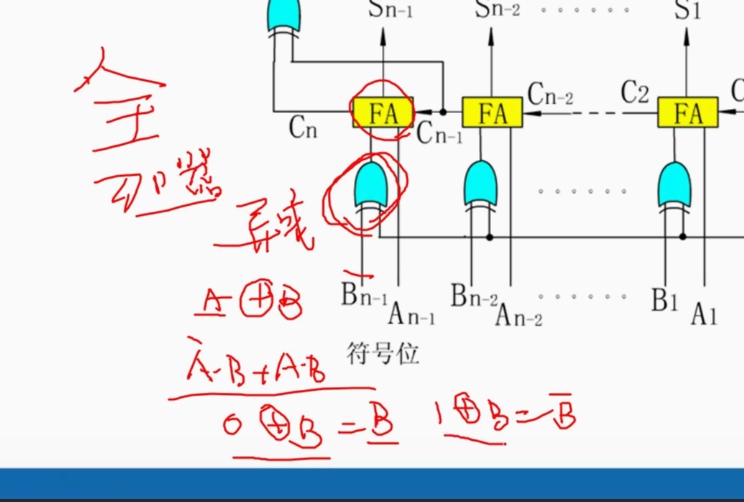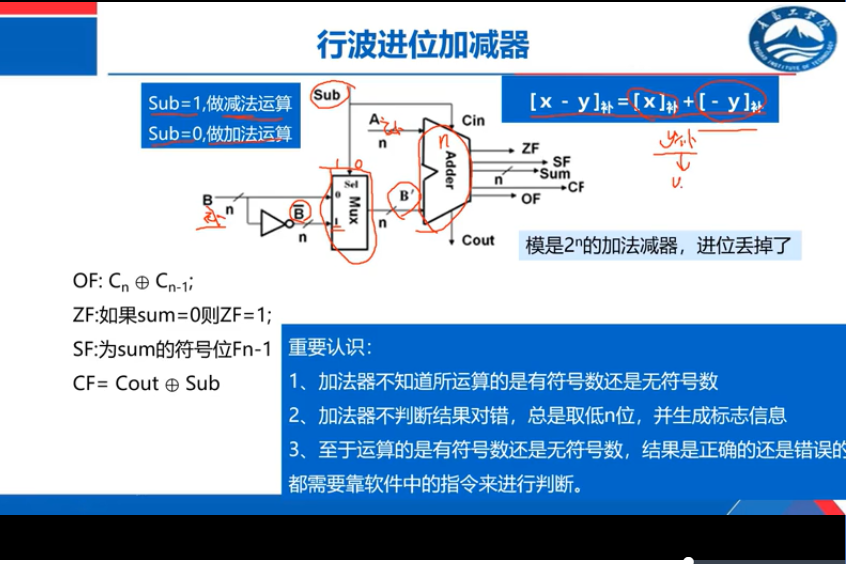Mux是多路选择器，当控制信号sub是0，将B送入，当控制信号sub为1，将B非送入。
在做减法时，需要-y的补码，通过公式，-y的补码就是y的补码连同符号位取反再加1，也就是y的非，在图上就是B非，加1传给进位标志位Cin。
Adder是加减器。
有符号整数加减法运算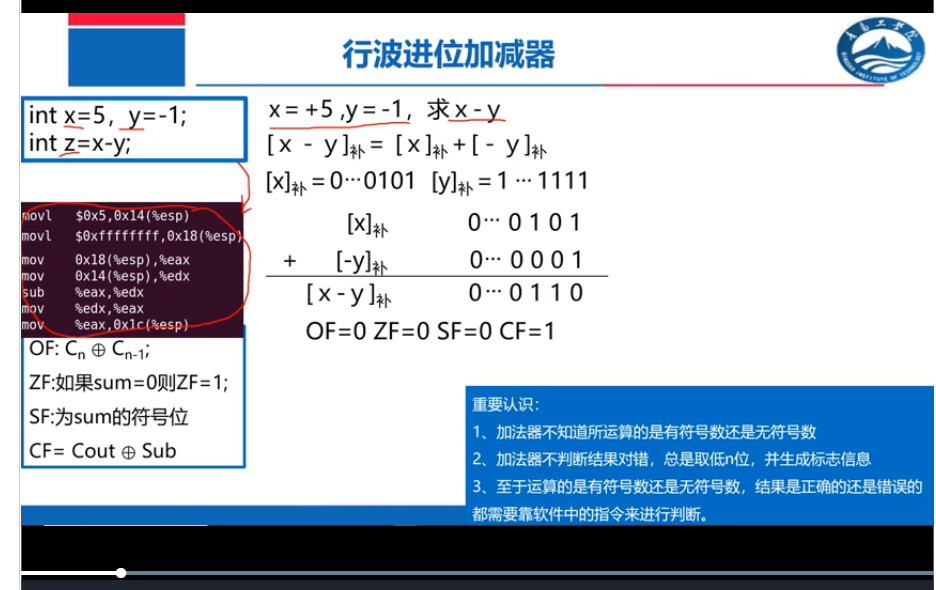将上面的C语言代码转换成汇编程序。SUB做减法运算。0x5是x，0x全f是-1.
将x和y变成补码是由编译器完成的。
sub指令最终是由加法实现的。由行波进位加减器实现。
sub指令被取入CPU之后，经过译码翻译出控制信号，这些控制信号会把两个操作数x送入A端，y送入B端。在sub端发出高电平信号，y最终是多少是由两路选择器的sel端来决定的。
由于sub为1，B‘端输出-1包括符号位取反，32个0，sub将1送入Cin，加减器实际上做的是三个数的加法，A，B’，1。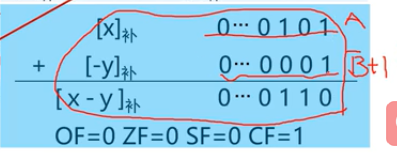溢出标志位OF：数值最高位无进位，符号最高位无进位，最后为0.
ZF零标志位，0 1 1 0四个数或非运算是0.
SF符号标志位就是符号运算最终结果0.
cf是Cout和Cin，Cin也就是sub，Cout是0，sub是1，最后是1。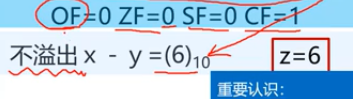最后输出的十进制 6是编译器转换后的结果，与行波无关。具体溢出不溢出也不是行波判断，它只是输出标志位，具体由编译器决定。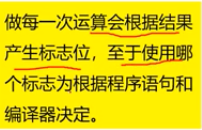有符号数使用的只是前三个标志位不使用CF。CF是无符号运算使用的。
无符号整数加减法运算无符号数不使用源码，补码，只使用一个二进制数表示。
具体转换也是编译器完成。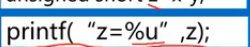假如C语言代码中由这样一条语句，要以无符号形式输出z，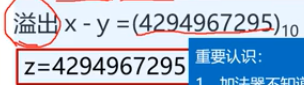编译器将每一位权值加起来转换成十进制，最后结果显示溢出，但是OF位是0.
无符号的特殊点是看是否溢出是由CF决定的。
无符号数的运算最后不看OF位，而是看CF位。
SF，OF没用。
四个标志位都会产生，但是编译器有选择地使用。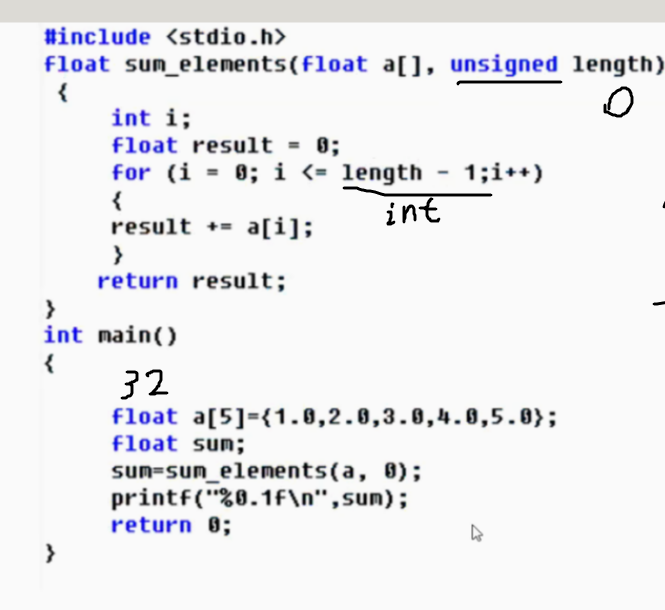无符号的0-1得到的是32个1转换成十进制非常大，result += a[i] 最终会发生数组越界错误。


展开全文• 来源：模板题 算法标签：高精度 题目描述： 给定两个正整数，计算它们的和。 输入格式 共两行，每行包含一个整数。 输出格式 共一行，包含所求的和。...2.逆序读写，模仿手动加法运算 3.t为进位，t+a[i]+b[i],即添...
来源：模板题
算法标签：高精度
题目描述：
给定两个正整数，计算它们的和。
输入格式
共两行，每行包含一个整数。
输出格式
共一行，包含所求的和。
数据范围
1≤整数长度≤100000
输入样例：
12
23
输出样例：
35
注意
len(a)<=10^6
len(b)<=10^6
思路
1.字符串存储A,B
2.逆序读写，模仿手动加法运算
3.t为进位，t+a[i]+b[i],即添加ab同列同位的两个数字，只增加%10，t/=10计算进位数目
4.完成，反序输出
模仿进程
模仿以下进程：
1.
12
23

5
3
35
2.
39
47

16
7

86

3.
aaa
bb

代码
#include<iostream>
#include<cstring>
#include<vector>

using namespace std;

vector<int> add(vector<int> &A,vector<int> &B)
{
if(A.size()<B.size())return add(B,A);//把长的那一部分置换为A，方便下面的计算

int t=0;//t进位
vector<int>C;//c ab和

for(int i=0;i<A.size();i++)//只要没走完长的部分
{
t+=A[i];//t加a位的数字
if(i<B.size())t+=B[i];//b还有数 +b[i]
C.push_back(t%10);//返回到C
t/=10;//进位
}

if(t)C.push_back(t);//如果还有余数，则加在最高位

return C;//返回
}

int main()
{
string a,b;
cin>>a>>b;

vector<int>A,B;
for(int i=a.size()-1;i>=0;i--)A.push_back(a[i]-'0');//逆序读入，返回数字
for(int i=b.size()-1;i>=0;i--)B.push_back(b[i]-'0');

vector<int>C = add(A,B);//c是a+b

for(int i=C.size()-1;i>=0;i--)cout<<C[i];//逆序输出

return 0;
}



展开全文• 还比较了业界常用的 7 种不同的算法模型, 使用 6 种不同指标对这些模型和多因素加法模型进.行评估, 实验结果发现, 在这 8 种不同算法模型中, 多因素加法模型有着更加精确的预测性能, 运算速度比其他.模型快, 并且...
• + 加法运算符 - 减法运算符，或负值运算符 * 乘法运算符 / 除法运算符 % 运算符，或称取余运算符，要求%两侧均为整型 2.关系运算符 < 小于运算符 <= ...
• 1.高精度加法模板 计算a+b， a与b长度均<=1e6 #include<iostream> #include<vector> using namespace std; vector<int> add(vector<int> &A,vector<int> &B) { int t=0; ...
• 加法运算： 复数的加法按照以下规定的法则进行：设z1=a+bi,z2=c+di是任意两个复数，则它们的和是(a+bi)+(c+di)=(a+c)+(b+d)i; 例如：a = 1+2i,b = 3+4i 即可得 a+b = 4+6i 减法法则： 复数的减法按照以下规定的法则...数据结构
• 一. 导入 0000 1110 —>14 1000 1110 ----> -14 1001 1100 -（4+8+16）= -28 ...所以，我们可以将减法转换为加法运算！ 二. 运算的性质 10-3=7 -3mod12=9 10+9=19 19/12=1…7即19mod12=7 带余除法
• 1.高精度加高精度（加法） 模拟小学算式 1 4 7 + 6 5 2 1 2 现在模拟这个算式即可从低位到高位每一位相加 7 + 5 = 12 这一位应该为2， 而进位则为12 / 10 = 1 4 + 6 + 1 = 11 这一位为1，而进位则为11 / 10 = 1； 1 ...
• 比较简短的高精度加法和减法运算模板。大除法的日后更新。 1 /* 两个非负的大整数相加 */ 2 void big_plus(char a[],char b[],char ans[]) 3 { 4 int c[N],d[N]; 5 memset(c,0,sizeof(c)); memset(d,0,...
• （5）取模运算符：用“%”表示，符号前的变量以符号后的变量为进行取模运算。取模运算是求两个数相除的余数 （6）求幂运算符：使用符号“**”表示，符号前的变量以符号后的变量为幂进行求幂运算。 （7）取整...python
• 如同以下表达式：x+5*y-(6/(1-5.5))可以表达为以下二叉树（抱歉，本来想弄Visualgo的，结果上不了，只能用word来做画面了）： 为什么是这样的二叉树呢？仔细想想平时是怎么计算这个表达式的，毫无疑问的是最后...
• 优先级从高到低的 1.分隔符（； （），{}） 小括号的优先级最高 2.单目运算符 ++ -- ~ ！ 3.强制类型转换符 type a =...6.位运算的 移位运算 > >>> 7.关系运算符的 比较运算符 > >= 8.关系运算符的等价符 == 和 !学习笔记
• 1. 引入补码的原因 在数字电路或系统中，为简化电路，常将负数用补码表示，以便将减法运算变为加法运算。以钟表为例，时间从6点钟减少到2点钟，可以通过6-4=2逆时针转动4个小时实现；也可以通过6+8=14(表盘为12进制...
• 算术运算符(7个)：+ - * / % ++ --，它们分别为：加法、减法、乘法、除法、求(也叫求余)、自增、自减。这里注意一点：在Golang中只支持后置++、后置–。 比较运算符(6个)：== != > < >= <=，它们分别...golang
• 1. 加法运算符 + 2. 减法运算符（负数运算符）- 3. 乘法运算符 * 4. 除法运算符 / 相同数据类型的值才能进行运算，而且运算结果依然是同一种数据类型 5. 运算符（取余运算符）% %两侧必须都为整数，否则不能运算 6...
• 1. 向量的基本概念1——向量、向量的表示、...4. 向量的线性运算加法和数乘，减法可以看做数乘与加法的复合） 5. 向量线性运算规则 6. 向量方程求解示例 7. 使用基向量表达向量（向量的线性表出） ...
• 1、对盒子模型的不同解释  这里IE6、IE8、IE9相同，火狐和谷歌、遨游等相同。...火狐谷歌等较新的的浏览器实行加法运算，“向外扩张”。以高度为例，CSS中设置的高度为内容的高度，当增大padding
• 1. 加法运算符 + 2. 减法运算符（负数运算符）- 3. 乘法运算符 * 4. 除法运算符 / 相同数据类型的值才能进行运算，而且运算结果依然是同一种数据类型 5. 运算符（取余运算符）% %两侧必须都为整数，否则不能运算 6...
• 也叫向量(数学中叫向量，物理中叫失量)，它有自己的方向和长，通常用来表示一个速度或是一个方向，有时候也会用来表示一个距离某点的距离。 失量的乘法、除法 各分量分别除以除数，意味着失量被按倍数放大或缩小，...矢量 向量的点乘和叉乘 Shader
• 2.大数加法、减法 3.大数乘大数 4.大数乘小数（高精度乘单精度） 5.大数除大数 6.大数除小数 7.快速幂运算 8.高精度开根 二、最短路 1.最短路1 2.最短路2 3.例题-昂贵的聘礼 三、最小生成树 1.最小生成...模板
• 实验内容：使用链式存储实现一元多项式的加法、减法、乘法和求导。即： C(x)= A(x)+B(x)；C(x)= A(x)-B(x) C(x)= A(x)*B(x) C(x)= A’(x) 菜单： 1)C ：分别创建两个多项式A(x)和B(x)，其中 输入时按照 指数的...
• 大整数类的四则运算1. 高精度加法2. 高精度减法3. 高精度和低精度的乘法★★★ 4. 高精度乘法5. 高精度与低精度的除法6. 高精度除法4. 题目和总结和预告 一道A+BA + BA+B的题目，都在intintint范围内，没有难度。...
• 在Mathematica之中多项式的基本运算包含加法，减法，乘法，除法，以及运算，值得注意的是多项式之后要加以分号分隔。。我们发现仅仅用除号使多项式运行，并不能使原有的多项式得到化简。如果我希望得到多项式的...
• 上一讲中鸡啄米创建了一个名为“Addition”的工程，目的是生成一个实现加法运算的应用程序。实现加法计算有几个必要的因素：被加数、加数、和。被加数和加数需要输入，和需要输出显示。那么这几个因素都......